As part of developing the Basel III framework, the Basel Committee on Banking Supervision initiated the Fundamental Review of the Trading Book (FRTB). Several years later, the outcome is a new set of global standards for calculating minimum capital requirements for market risk in the trading book. The EU plans to implement the standards for European credit institutions in the Capital Requirements Regulation/Directive (CRR/CRD) in the coming years.

In this blog, we investigate Danish fixed-rate callable mortgage bonds (callables) which is the largest sub-group in the Danish mortgage bond market. The underlying loans are prepayable which implies that the borrower may redeem his or her loan at par value. The prepayment option results in a non-linear relationship between price and interest changes and therefore the bond is subject to curvature and vega capital requirements.

Danish banks are not among the main investors for these types of bonds. In October 2019, out of a total outstanding amount of 1,176 bn DKK, Danish banks hold only about 90 bn DKK1.

In this blog we show that the two new risk measures introduced by FRTB, curvature and vega, increase the GIRR capital charges for callables substantially. Low coupon bonds with modest prepayment risk are sensitive to volatility changes, while high coupon bonds with considerable prepayment risk have high curvature risk components. The increase in capital requirements makes it more important than ever for banks to correctly hedge callables in the trading book.

This blog is part of our series where we investigate FRTB’s impact on capital requirements for Danish bonds. You might also want to read

## Setting the stage for SA-FRTB calculations

As discussed in previous blogs, we are interested in the two risk classes, GIRR and CSR, as well as the three risk measures delta, curvature and vega. In the CSR risk class, only delta is relevant because there are no embedded options on the issuer credit spread. The GIRR risk class is, however, extended with a new set of risk factors related to the refinancing curve.

Danish callable mortgage bonds are predominantly modelled using two yield curves

• Like most fixed-income instruments, the cash flow is discounted based on a yield curve estimated from money market instruments like FRAs and swaps. An option-adjusted spread is often added to the discounting.
• The cash flow itself depends on borrower prepayment behavior and is therefore stochastic. The borrower decides whether to prepay his loan based on the refinancing rates available in the loan market.

We already know that the discounting curve belongs to GIRR, but what about the refinancing curve? We will answer that question later in the blog, but first let us have a look at how the refinancing curve is constructed in our models.

### The refinancing curve

We construct the refinancing curve as the Danish Cibor-6M swap curve with an added refinancing spread estimated from observed yield pickups of open callable series. We employ a method where today’s level of the refinancing spread is used to calculate short term refinancing gains, e.g. for payment dates shorter than three years. In our model, this short-term level is gradually reverted towards a stable, long term level when calculating longer term gains. The refinancing curve based on the long-term refinancing spread is shown in Figure 1.Figure 1: The Danish Cibor-6M swap curve on 21 October 2019 and the refinancing curve using a refinancing spread of 85 bps.

Figure 2 shows how the price of callables depends on the refinancing rates. It shows that when refinancing becomes more expensive, i.e. the rates increase, the prepayment option loses value and the price of the bond converges towards the non-callable bond price.Figure 2: The price dependency of refinancing rates for “2% NYK 2050” on 21 October 2019. Only refinancing rates are shifted meaning that the discounting rates are fixed. The bond has a market price of 103,198. The dotted line represents the non-callable bond price.

The relationship between discount rates and refinancing rates can also be illustrated by comparing expected cash flows under different yield curve scenarios. Below, we consider “2% JYK 2047 IO” (DK0009387938) which has high prepayment risk. The expected cash flow with unchanged yield curves is shown in Figure 3.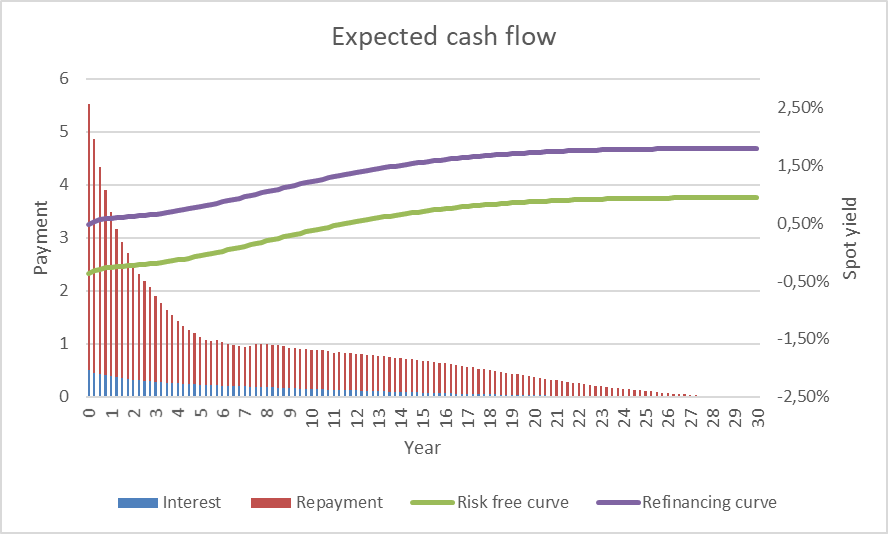Figure 3: Expected cash flow of 2% JYK 2047 IO.

When the refinancing curve is shifted up and down, the expected cash flow changes. This is shown in Figure 4 and Figure 5.Figure 4: Expected cash flow of 2% JYK 2047 IO for lowered refinancing rates. Almost all borrowers decide to prepay their loans and the option adjusted duration of the bond is very low.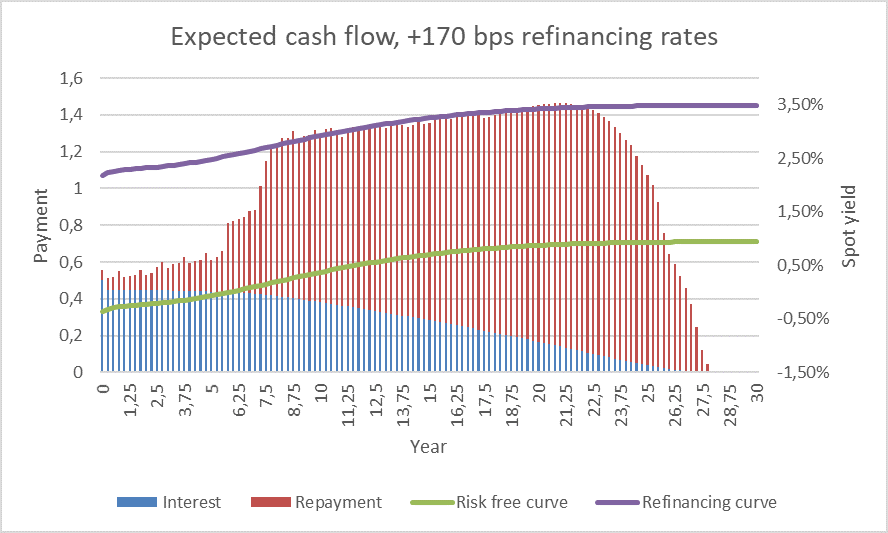Figure 5: Expected cash flow of 2% JYK 2047 IO for increased refinancing rates. Prepayment is less attractive, and the cash flow resembles a regular deferred annuity.

On the other hand, if discount rates are shifted up and down, the cash flow is unchanged while the present value of the payments changes. This is shown in Figure 6 and Figure 7.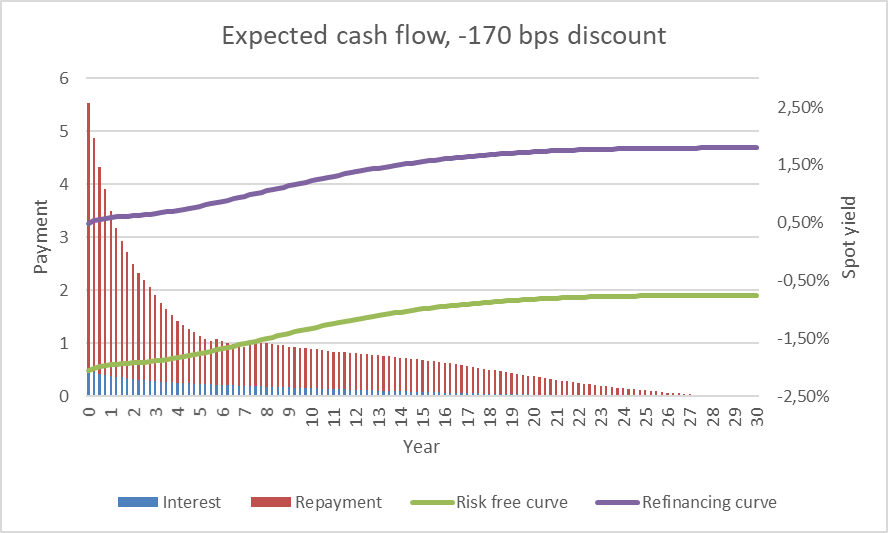Figure 6: Expected cash flow of 2% JYK 2047 IO for lowered discount rates. The present value of the cash flow increases compared to Figure 3.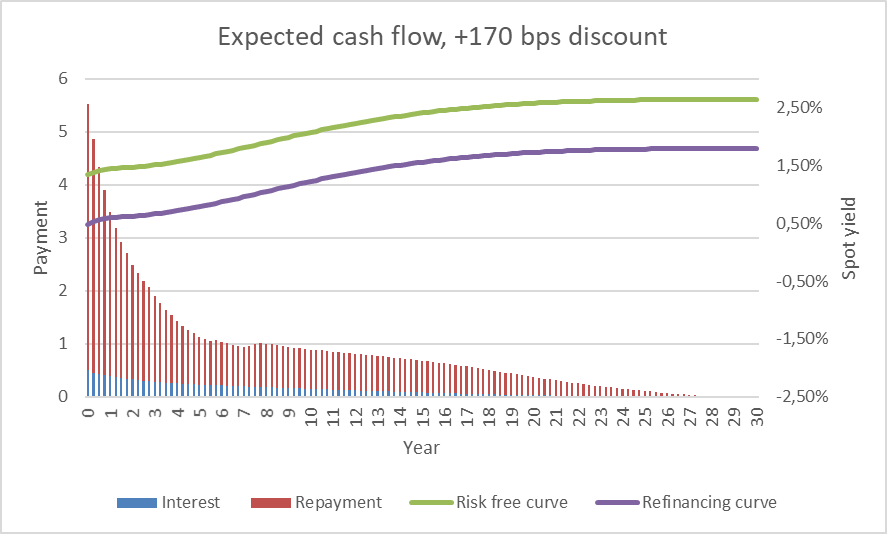Figure 7: Expected cash flow of 2% JYK 2047 IO for increased discount rates. The present value of the cash flow decreases compared to Figure 3.

What kind of risk factor is the refinancing curve?

Refinancing curves are not explicitly mentioned in Basel (2019). Based on the above, we identify two risk factors in the refinancing curve. First, we have the CIBOR6M curve which is classified as a risk-free yield curve belonging to GIRR. Second, we have the refinancing spread which is a credit spread and hence belonging to CSR. That leaves us with the following list of FRTB risk factors for callables.

CLASS MEASURE BUCKET FACTORS REFERENCE
GIRR Delta Currency  Risk-free yield curves @
0.25, 0.5, 1, 2, 3, 5, 10, 15, 20, 30Y

21.8.(1)

CSR Delta Rating and sector Issuer credit spread curve @ 0.5, 1, 3, 5, 10Y
Borrower’s credit spread curve @ 0.5, 1, 3, 5, 10Y
21.9.(1)
GIRR Curvature Currency Risk-free yield curve (no term structure decomposition) 21.8.(5)
GIRR Vega Currency  Implicit volatilities of interest rate options defined along two dimensions: The maturity of the option and the maturity of the underlying, both @ 0.5, 1, 3, 5, 10Y 21.8.(4)

Table 1: Risk factors related to Danish callable mortgage bonds defined by FRTB. Reference paragraphs refer to Basel (Jan. 2019).

### Capital impact, GIRR is the main driver

Table 2 shows the list of callables we will use in the impact analysis. All calculations are performed on a non-hedged single bond portfolio.

ISIN NAME LAST PAY DATE CAPITAL CENTER INTEREST ONLY OUTSTANDING AMOUNT
DK0004612025 0.5% RD 2035 01-10-2035 S 20,22 4,12
DK0009504326 1.5% NYK 2037 01-10-2037 E 15,44  15,92
DK0009524357 0.5% NYK 2040 01-10-2040 E 13,30  12,96
DK0009515017 1% NYK 2040 01-10-2040 E 3,17 9,47
DK0009525081 0.5% NYK 2050 01-10-2050 E 0,86 16,02
DK0009525321 0.5% NYK 2050 IO 01-10-2050 E 4,01 6,17
DK0009522815 1% NYK 2050 01-10-2050 E 11,08 53,31
DK0009524431 1% NYK 2050 IO 01-10-2050 E 1,20 52,83
DK0009513152 1.5% NYK 2050 01-10-2050 E 2,52 38,69
DK0009513582 2% NYK 2050 01-10-2050 E 2,52 52,82
DK0009392854 2% JYK 2050 IO 01-10-2050 E 2,52 6,55

Table 2: Danish fixed rate callable mortgage bonds. Outst.amount is in billion DKK and from 21 October 2019.

GIRR Delta

The GIRR delta vector for 2% NYK 2050 is shown in Table 3. The bond has high prepayment risk and low option adjusted duration. It is the sensitivity to the refinancing curve that drives the duration down.

CURVE KEY RATE SENSITIVITY ($$s_k$$) RISK WEIGHT ($$RW_{k}$$*) WEIGHTED SENSITIVITY ($$WS_{k}$$)
0.25 0.0569 1.7% / $$\sqrt{2}$$ 0.0684
0.50 0.0877 1.7% / $$\sqrt{2}$$ 0.1055
DKKCITA  1 0.1920 1.6% / $$\sqrt{2}$$ 0.2172
(discount) 2 0.2168 1.3% / $$\sqrt{2}$$ 0.1993
3 0.2362 1.2% / $$\sqrt{2}$$ 0.2005
5 0.5237 1.1% / $$\sqrt{2}$$ 0.4073
10 0.7126 1.1% / $$\sqrt{2}$$ 0.5543
15 0.6371 1.1% / $$\sqrt{2}$$ 0.4955
20 0.7374 1.1% / $$\sqrt{2}$$ 0.5736
30 0.3451 1.1% / $$\sqrt{2}$$ 0.2684
0.25 0.0065 1.7% / $$\sqrt{2}$$ 0.0078
0.50 0.0256 1.7% / $$\sqrt{2}$$ 0.0308
1 0.0549 1.6% / $$\sqrt{2}$$ 0.0621
DKK CIBOR6M 2 0.0570 1.3% / $$\sqrt{2}$$ 0.0524
(refinancing) 3 0.0349 1.2% / $$\sqrt{2}$$ 0.0296
5 -0.0537 1.1% / $$\sqrt{2}$$ -0.0418
10 -0.3218 1.1% / $$\sqrt{2}$$ -0.2503
15 -0.5735 1.1% / $$\sqrt{2}$$ -0.4461
20 -1.2680 1.1% / $$\sqrt{2}$$ -0.9862
30 -0.9124 1.1% / $$\sqrt{2}$$ -0.7096

Table 3: GIRR delta sensitivities for ”2% NYK 2050” on 21 October 2019. The sensitivities are expressed for a 1 pct. shift size. The bond has a high coupon compared to the prevailing mortgage loan rates. The bond value increases when long-term refinancing rates increase.

The GIRR delta capital charge is calculated in the same way as in the previous blogs.

$$K^{Delta}_{GIRR}= \sqrt{\Bigl(\sum_{k}\sum_{l}\rho_{kl}WS_kWS_l\Bigr)^+}$$

Where $$\rho_{kl}$$ is a correlation in a 20x20 correlation matrix.

GIRR Curvature and vega

When calculating the GIRR curvature capital charge, the discounting and refinancing curves are shifted simultaneously. The calculations have already been explained in our blog about floaters.

We will not delve deep into the vega calculations in this blog. We are using a preliminary version of a vega decomposition and have not applied correlations between the vega risk factors.

### Results

Figure 8 shows the capital impacts on a single bond level. For most of the bonds, the capital requirements more than doubles. The longest bonds are affected the most, but the coupon rate also plays a major role. In October 2019 with refinancing rates around 1%, the prepayment options of the loans funded in the 2% 2050 series are in the money and prepayment is very likely. The duration of the bonds is therefore next to zero, and in the current legislation the capital requirements imposed on the bonds are quite small.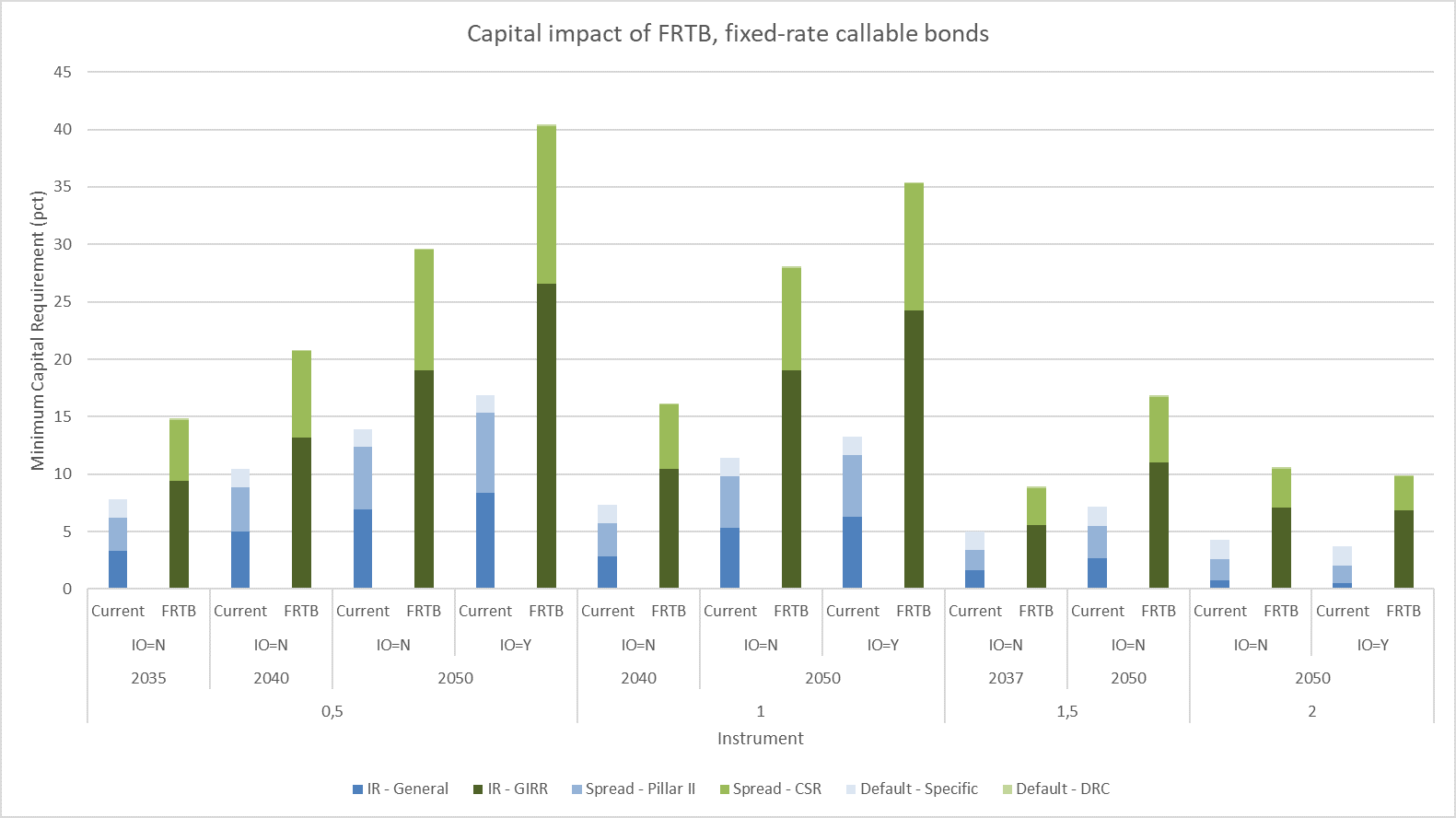Figure 8: Estimated capital impact of Danish callable mortgage bonds for Danish banks. We estimate that the capital requirements increase with more than 100 percent for several of the bonds.

But even if a 2% 2050 callable bond has a duration of about zero, it can still incorporate significant interest rate risk. If interest rates start to increase, the price of such bonds can drop rapidly and cause substantial losses to the bank. Figure 9 shows the GIRR risk class decomposed into the three different risk measures.Figure 9: GIRR decomposed into delta, curvature and vega for callabes. The numbers are compared to current legislation which only considers first-order interest rate risk. Long-term bonds with low prepayment risk are rather sensitive to changes in volatility, while bonds with high prepayment risk are mainly capitalized due to the second-order interest rate risk component (curvature).

The vega component is substantial for low coupon bonds. When volatility increases, the prepayment option becomes more valuable driving bond prices down. Contrary to RTL bonds and floating rate notes, the CSR component is not the main source of the capital increase. The GIRR component grows substantially because of the two new risk measures, curvature and vega, introduced by FRTB.

## Key takeaways

Of the three main bond types in the Danish mortgage bond market, fixed rate callable mortgage bonds are faced with the largest capital charges as a result of FRTB. The bonds used for funding new mortgage loans having 30 years to maturity and low prepayment risk face an estimated capital charge of 30% of their market value for regular annuities and 40% for deferred annuities. This is more than twice the current level, and the increase is mainly driven by the new curvature and vega risk measures in the GIRR risk class.

Danish banks currently have a low demand for such bonds as they hold only about 4% of the total outstanding, and the increased capital charges imposed by FRTB are not likely to increase the banks’ investment appetite.

This blog concludes our series of FRTB impact analyses. Please contact Scanrate at support@scanrate.dk (phone +45 86 205 210) if you have questions on this topic or if you are interested in hearing about our solutions for FRTB compliance.

Footnote:

1 The main investors in Danish callable mortgage bonds are pension and investment funds. Source: https://nationalbanken.statistikbank.dk/.

Referencer:

- Basel Committee on Banking Supervision, January 2019: Minimum capital requirements for market risk,
https://www.bis.org/bcbs/publ/d457.htm

Figure 1: The Danish Cibor-6M swap curve on 21 October 2019 and the refinancing curve using a refinancing spread of 85 bps.

Figure 2 shows how the price of callables depends on the refinancing rates. It shows that when refinancing becomes more expensive, i.e. the rates increase, the prepayment option loses value and the price of the bond converges towards the non-callable bond price.Figure 2: The price dependency of refinancing rates for “2% NYK 2050” on 21 October 2019. Only refinancing rates are shifted meaning that the discounting rates are fixed. The bond has a market price of 103,198. The dotted line represents the non-callable bond price.

The relationship between discount rates and refinancing rates can also be illustrated by comparing expected cash flows under different yield curve scenarios. Below, we consider “2% JYK 2047 IO” (DK0009387938) which has high prepayment risk. The expected cash flow with unchanged yield curves is shown in Figure 3.Figure 3: Expected cash flow of 2% JYK 2047 IO.

When the refinancing curve is shifted up and down, the expected cash flow changes. This is shown in Figure 4 and Figure 5.Figure 4: Expected cash flow of 2% JYK 2047 IO for lowered refinancing rates. Almost all borrowers decide to prepay their loans and the option adjusted duration of the bond is very low.Figure 5: Expected cash flow of 2% JYK 2047 IO for increased refinancing rates. Prepayment is less attractive, and the cash flow resembles a regular deferred annuity.

On the other hand, if discount rates are shifted up and down, the cash flow is unchanged while the present value of the payments changes. This is shown in Figure 6 and Figure 7.Figure 6: Expected cash flow of 2% JYK 2047 IO for lowered discount rates. The present value of the cash flow increases compared to Figure 3.Figure 7: Expected cash flow of 2% JYK 2047 IO for increased discount rates. The present value of the cash flow decreases compared to Figure 3.

What kind of risk factor is the refinancing curve?

Refinancing curves are not explicitly mentioned in Basel (2019). Based on the above, we identify two risk factors in the refinancing curve. First, we have the CIBOR6M curve which is classified as a risk-free yield curve belonging to GIRR. Second, we have the refinancing spread which is a credit spread and hence belonging to CSR. That leaves us with the following list of FRTB risk factors for callables.

CLASS MEASURE BUCKET FACTORS REFERENCE
GIRR Delta Currency  Risk-free yield curves @
0.25, 0.5, 1, 2, 3, 5, 10, 15, 20, 30Y

21.8.(1)

CSR Delta Rating and sector Issuer credit spread curve @ 0.5, 1, 3, 5, 10Y
Borrower’s credit spread curve @ 0.5, 1, 3, 5, 10Y
21.9.(1)
GIRR Curvature Currency Risk-free yield curve (no term structure decomposition) 21.8.(5)
GIRR Vega Currency  Implicit volatilities of interest rate options defined along two dimensions: The maturity of the option and the maturity of the underlying, both @ 0.5, 1, 3, 5, 10Y 21.8.(4)

Table 1: Risk factors related to Danish callable mortgage bonds defined by FRTB. Reference paragraphs refer to Basel (Jan. 2019).

### Capital impact, GIRR is the main driver

Table 2 shows the list of callables we will use in the impact analysis. All calculations are performed on a non-hedged single bond portfolio.

ISIN NAME LAST PAY DATE CAPITAL CENTER INTEREST ONLY OUTSTANDING AMOUNT
DK0004612025 0.5% RD 2035 01-10-2035 S 20,22 4,12
DK0009504326 1.5% NYK 2037 01-10-2037 E 15,44  15,92
DK0009524357 0.5% NYK 2040 01-10-2040 E 13,30  12,96
DK0009515017 1% NYK 2040 01-10-2040 E 3,17 9,47
DK0009525081 0.5% NYK 2050 01-10-2050 E 0,86 16,02
DK0009525321 0.5% NYK 2050 IO 01-10-2050 E 4,01 6,17
DK0009522815 1% NYK 2050 01-10-2050 E 11,08 53,31
DK0009524431 1% NYK 2050 IO 01-10-2050 E 1,20 52,83
DK0009513152 1.5% NYK 2050 01-10-2050 E 2,52 38,69
DK0009513582 2% NYK 2050 01-10-2050 E 2,52 52,82
DK0009392854 2% JYK 2050 IO 01-10-2050 E 2,52 6,55

Table 2: Danish fixed rate callable mortgage bonds. Outst.amount is in billion DKK and from 21 October 2019.

GIRR Delta

The GIRR delta vector for 2% NYK 2050 is shown in Table 3. The bond has high prepayment risk and low option adjusted duration. It is the sensitivity to the refinancing curve that drives the duration down.

CURVE KEY RATE SENSITIVITY ($$s_k$$) RISK WEIGHT ($$RW_{k}$$*) WEIGHTED SENSITIVITY ($$WS_{k}$$)
0.25 0.0569 1.7% / $$\sqrt{2}$$ 0.0684
0.50 0.0877 1.7% / $$\sqrt{2}$$ 0.1055
DKKCITA  1 0.1920 1.6% / $$\sqrt{2}$$ 0.2172
(discount) 2 0.2168 1.3% / $$\sqrt{2}$$ 0.1993
3 0.2362 1.2% / $$\sqrt{2}$$ 0.2005
5 0.5237 1.1% / $$\sqrt{2}$$ 0.4073
10 0.7126 1.1% / $$\sqrt{2}$$ 0.5543
15 0.6371 1.1% / $$\sqrt{2}$$ 0.4955
20 0.7374 1.1% / $$\sqrt{2}$$ 0.5736
30 0.3451 1.1% / $$\sqrt{2}$$ 0.2684
0.25 0.0065 1.7% / $$\sqrt{2}$$ 0.0078
0.50 0.0256 1.7% / $$\sqrt{2}$$ 0.0308
1 0.0549 1.6% / $$\sqrt{2}$$ 0.0621
DKK CIBOR6M 2 0.0570 1.3% / $$\sqrt{2}$$ 0.0524
(refinancing) 3 0.0349 1.2% / $$\sqrt{2}$$ 0.0296
5 -0.0537 1.1% / $$\sqrt{2}$$ -0.0418
10 -0.3218 1.1% / $$\sqrt{2}$$ -0.2503
15 -0.5735 1.1% / $$\sqrt{2}$$ -0.4461
20 -1.2680 1.1% / $$\sqrt{2}$$ -0.9862
30 -0.9124 1.1% / $$\sqrt{2}$$ -0.7096

Table 3: GIRR delta sensitivities for ”2% NYK 2050” on 21 October 2019. The sensitivities are expressed for a 1 pct. shift size. The bond has a high coupon compared to the prevailing mortgage loan rates. The bond value increases when long-term refinancing rates increase.

The GIRR delta capital charge is calculated in the same way as in the previous blogs.

$$K^{Delta}_{GIRR}= \sqrt{\Bigl(\sum_{k}\sum_{l}\rho_{kl}WS_kWS_l\Bigr)^+}$$

Where $$\rho_{kl}$$ is a correlation in a 20x20 correlation matrix.

GIRR Curvature and vega

When calculating the GIRR curvature capital charge, the discounting and refinancing curves are shifted simultaneously. The calculations have already been explained in our blog about floaters.

We will not delve deep into the vega calculations in this blog. We are using a preliminary version of a vega decomposition and have not applied correlations between the vega risk factors.

### Results

Figure 8 shows the capital impacts on a single bond level. For most of the bonds, the capital requirements more than doubles. The longest bonds are affected the most, but the coupon rate also plays a major role. In October 2019 with refinancing rates around 1%, the prepayment options of the loans funded in the 2% 2050 series are in the money and prepayment is very likely. The duration of the bonds is therefore next to zero, and in the current legislation the capital requirements imposed on the bonds are quite small.Figure 8: Estimated capital impact of Danish callable mortgage bonds for Danish banks. We estimate that the capital requirements increase with more than 100 percent for several of the bonds.

But even if a 2% 2050 callable bond has a duration of about zero, it can still incorporate significant interest rate risk. If interest rates start to increase, the price of such bonds can drop rapidly and cause substantial losses to the bank. Figure 9 shows the GIRR risk class decomposed into the three different risk measures.Figure 9: GIRR decomposed into delta, curvature and vega for callabes. The numbers are compared to current legislation which only considers first-order interest rate risk. Long-term bonds with low prepayment risk are rather sensitive to changes in volatility, while bonds with high prepayment risk are mainly capitalized due to the second-order interest rate risk component (curvature).

The vega component is substantial for low coupon bonds. When volatility increases, the prepayment option becomes more valuable driving bond prices down. Contrary to RTL bonds and floating rate notes, the CSR component is not the main source of the capital increase. The GIRR component grows substantially because of the two new risk measures, curvature and vega, introduced by FRTB.

## Key takeaways

Of the three main bond types in the Danish mortgage bond market, fixed rate callable mortgage bonds are faced with the largest capital charges as a result of FRTB. The bonds used for funding new mortgage loans having 30 years to maturity and low prepayment risk face an estimated capital charge of 30% of their market value for regular annuities and 40% for deferred annuities. This is more than twice the current level, and the increase is mainly driven by the new curvature and vega risk measures in the GIRR risk class.

Danish banks currently have a low demand for such bonds as they hold only about 4% of the total outstanding, and the increased capital charges imposed by FRTB are not likely to increase the banks’ investment appetite.

This blog concludes our series of FRTB impact analyses. Please contact Scanrate at support@scanrate.dk (phone +45 86 205 210) if you have questions on this topic or if you are interested in hearing about our solutions for FRTB compliance.

Footnote:

1 The main investors in Danish callable mortgage bonds are pension and investment funds. Source: https://nationalbanken.statistikbank.dk/.

Referencer:

- Basel Committee on Banking Supervision, January 2019: Minimum capital requirements for market risk,
https://www.bis.org/bcbs/publ/d457.htm